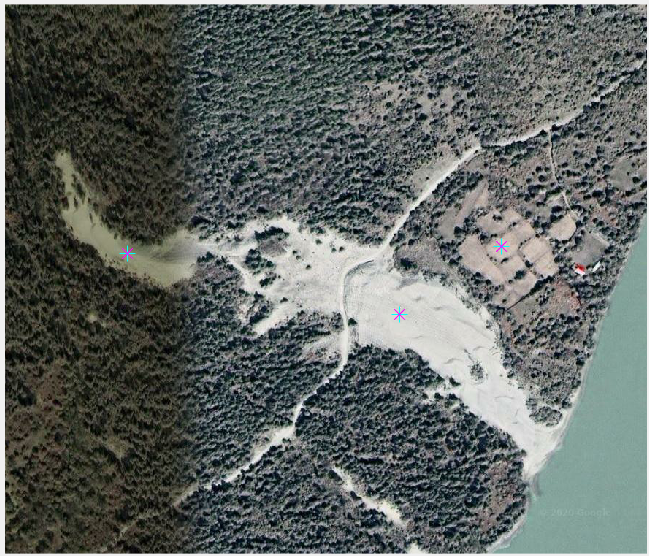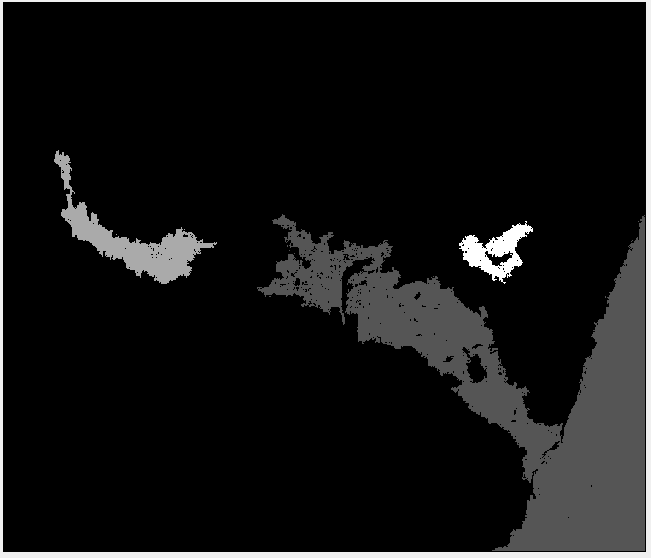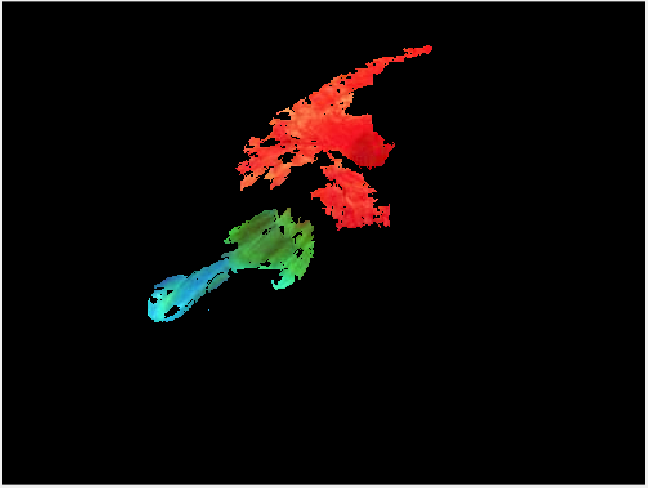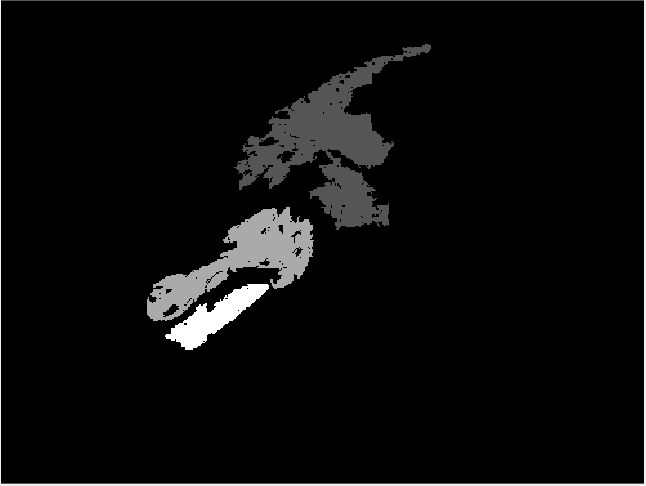• Matlab边缘检测和区域生长图像分割算法代码-regiongrow.m 我的毕业设计，顺利通过。希望对朋友们有帮助。matlab
• 根据pcnn的特性，将其与区域生长算法结合，完成图像分割
• 5行行Python代代码码实实现现图图像像分分割割的的步步骤骤详详解解 这篇文章主要介绍了5行Python代码实现图像分割的步骤详解文中通过示例代码介绍的非常详细对大家的学习 或者工作具有 定的参考学习价值需要的朋友...
• 基于区域相似的图像分割 matlab的区域生长算法
• 基于区域生长法的图像分割matlab代码明显的结果 该存储库中的代码是Shih和Cheng撰写的论文“用于彩色图像分割的自动播种区域生长”的MATLAB实现。 该方法包括4个主要部分： 将RGB图像转换为YCbCr颜色空间 自动选种 ...
• Matlab边缘检测和区域生长图像分割算法代码-deer.m 我的毕业设计，顺利通过。希望对朋友们有帮助。matlab
• 基于区域生长图像分割，可以自己设置区域生长点。区域生长是一种串行区域分割图像分割方法。区域生长是指从某个像素出发，按照一定的准则，逐步加入邻近像素，当满足一定的条件时，区域生长终止。
• 算法流程： 选取种子点p(x0，y0)，用堆栈表示种子区域，将种子点push到种子堆栈中； 将种子堆栈中第一个种子点pop出堆栈，并以该点为中心，遍历该中心8邻域像素； 判断遍历像素点是否已经在种子区域中 3.1 是，...

时间：2020-12-07

1. 流程简述
算法流程：
选取种子点p(x0，y0)，用堆栈表示种子区域，将种子点push到种子堆栈中；将种子堆栈中第一个种子点pop出堆栈，并以该点为中心，遍历该中心8邻域像素；判断遍历像素点是否已经在种子区域中 3.1 是，continue； 3.2 否，检查点p(x,y)和种子区域的均值refAvg灰度差是否满足阈值；若满足，将p(x, y) push到堆栈中；若不满足，continue；计算新的种子区域的均值refAvg，找出离上一个种子"最近"的seed，更新堆栈顺序；重复步骤 2-3-4，直至种子堆栈为空。
3. 源码
源码v1.0，目前存在的问题是：
生长过程中，以下代码只会寻找下一个种子（仅一个），取决于迭代的顺序。最终，可能导致分割效果不同。  另一个问题是阈值的选择问题。
修改策略：自适应阈值？全局迭代？后续补充v2.0。
int negihborRegionCheck(
unsigned char   *pSrc,
unsigned char   *pDst,
seedInfo_t      *seedPts,
unsigned int    *seedCnt,
imageSize_t     srcSize,
unsigned char   threshold
) {

if (nullptr == pSrc || nullptr == pDst || nullptr == seedPts || nullptr == seedCnt ||
srcSize.width <= 0 || srcSize.height <= 0) {
// input err.
return -1;
}

// malloc buffer for seed list.
seedInfo_t *listSeedPts = (seedInfo_t *)malloc(sizeof(seedInfo_t) * MAX_SEED_NUM);

// get seedPts[]
memcpy(listSeedPts, seedPts, sizeof(seedInfo_t) * (*seedCnt));

// get 1-st seed pts.
int numRegSeedList = *seedCnt;

int resBoundary = 0;
int nbPts = { {-1, -1},
{ 0, -1},
{ 1, -1},
{-1,  0},
{ 1,  0},
{-1,  1},
{ 0,  1},
{ 1,  1} };

// get region mean.
float refAvg = listSeedPts.var;

while (numRegSeedList > 0) {
#ifdef ENABLE_OPENCV
cv::Mat ori(srcSize.height, srcSize.width, CV_8UC1, (void *)pSrc);
cv::Mat dst(srcSize.height, srcSize.width, CV_8UC1, (void *)pDst);
#endif
// pop a seed.
int x = listSeedPts.pts.x;
int y = listSeedPts.pts.y;
pDst[y * srcSize.width + x] = 255;  // mark seed.

// iter stack.
for (int k = numRegSeedList - 1; k > 0; k--) {
listSeedPts[k - 1] = listSeedPts[k];
}

listSeedPts[numRegSeedList - 1].pts.x = 0;
listSeedPts[numRegSeedList - 1].pts.y = 0;
listSeedPts[numRegSeedList - 1].var = 0;

numRegSeedList -= 1;

for (int i = 0; i < 8; i++) {
unsigned int xn = x + nbPts[i];
unsigned int yn = y + nbPts[i];

resBoundary = ((xn >= 0) && (xn <= srcSize.width - 1) &&
(yn >= 0) && (yn <= srcSize.height - 1));

if (resBoundary && pDst[yn * srcSize.width + xn] == 0) {
if (fabs(pSrc[yn * srcSize.width + xn] - refAvg) <= threshold) {
pDst[yn * srcSize.width + xn] = 255;

// add seed to list, push pts in stack.
listSeedPts[numRegSeedList].pts.x = xn;
listSeedPts[numRegSeedList].pts.y = yn;
listSeedPts[numRegSeedList].var = pSrc[yn * srcSize.width + xn];

numRegSeedList += 1;
}
}
}

// find next seed.
int idx = 0;
int minVar = 255;
float sumReg = pSrc[y * srcSize.width + x];

for (int i = 0; i < numRegSeedList; i++) {
if (minVar >= fabs(pSrc[y * srcSize.width + x] - listSeedPts[i].var)) {
minVar = fabs(pSrc[y * srcSize.width + x] - listSeedPts[i].var);
idx = i;
}
sumReg += listSeedPts[i].var;
}

if (sumReg > 0 && numRegSeedList > 0) {
refAvg = sumReg / (numRegSeedList + 1);
}

// update list (swap element).
if (idx > 0) {
int tempVar = listSeedPts.var;
int tempX = listSeedPts.pts.x;
int tempY = listSeedPts.pts.y;

listSeedPts.var = listSeedPts[idx].var;
listSeedPts.pts.x = listSeedPts[idx].pts.x;
listSeedPts.pts.y = listSeedPts[idx].pts.y;

listSeedPts[idx].var = tempVar;
listSeedPts[idx].pts.x = tempX;
listSeedPts[idx].pts.y = tempY;
}
}

if (listSeedPts) {
free(listSeedPts);
}

return 0;
}

展开全文• 区域生长及其实现(matlab)

影像分割

区域生长及其实现
区域生长是根据事先定义的准则将像素或者子区域聚合城更大区域的过程。 其基本思想 是从一组生长点开始（生长点可以是单个像素， 也可以为某个小区域），将与该生长点性质相 似的相邻像素或者区域与生长点合井， 形成新的生长点， 重复此过程直到不能生长为止。 生 长点和相邻区域的相似性判据可以是灰度值、 纹理、 颜色等多种图像信息。
区域生长算法 区域生长一般有3个步骤。 (1) 选择合适的生长点。 (2) 确定相似性准则即生长准则 (3) 确定生长停止条件。 一般来说， 在无像素或者区域不满足加入生长区域的条件时， 区域生长就会停止。
function Image1 = myregionGrow(Image)
[m,n,band]=size(Image);
Image=double(Image);
[y,x]=getpts;
T=6;%设置阈值,这个根据需要改变
L=3;
Point=[x;y];%选择的点
Point=reshape(Point,size(Point,1)/2,2);%重塑矩阵，方便获取坐标
[M,~]=size(Point);%获取行数，作为序号
Image1=zeros(m,n,band);
for i=1:M %对所获取的坐标点分别生成起始种子
x=Point(i,1);%获取种子的x坐标，y坐标
y=Point(i,2);
k=i*255/M;%给和种子相似的区域赋值指定的值
newseed=[x,y];
while(size(newseed,1)>0)
t=[];%临时变量存储新生长的部分
for p=1:size(newseed,1)%对当前种子进行循环遍历
x=round(newseed(p,1));%获取x坐标
y=round(newseed(p,2));%获取y坐标
%搜索种子周围25个点
if( x>L && x<=m-L && y>L && y<=n-L)%判断是否超过图像边界
for u = -L:L
for v = -L:L
X = x + u; Y = y + v;
if band==3
R=Image(X,Y,1);r=Image(x,y,1);
G=Image(X,Y,2);g=Image(x,y,2);
B=Image(X,Y,3);b=Image(x,y,3);
if(abs(R-r)<=T&&abs(G-g)<=T&&abs(B-b)<=T && Image1(X,Y)==0)
for s=1:3
Image1(X,Y,s) = k;%给满足条件的点做上标记，特定灰度值
%                           Image1(X,Y,s)=Image(X,Y,s);%保存原来颜色
end
t=[t;X,Y];%新坐标增加到临时变量中
end
elseif band==1
if( X>1 && X<m && Y>1 && Y<n && abs(Image(X,Y,1)-Image(x,y,1))<=T && Image1(X,Y)==0)
Image1(X,Y) = k;%给满足条件的点做上标记，特定灰度值
t=[t;X,Y];%新坐标增加到临时变量中
end
end
end
end
end
end
newseed=t;%新生长区域作为新种子
end
end
end

滑坡影像测试：（图中米字型标点为选择的点）测试结果：（黑色为背景色，根据代码给图像赋值具有区分度的灰度值，当然也可以保留原有颜色）原图：处理后赋值色彩：处理后赋值灰度：可以看出，最后分割结果可能与设置的阈值和搜索距离有关，当距离或者阈值太大可能导致合并现象，及多种目标合并成为一种。
参考文献：数字图像处理与机器视觉visual C++与matlab实现 和CSDN上各种文章
展开全文计算机视觉
• 为克服一般区域生长算法对初始种子点选择以及生长顺序鲁棒性较差的问题,提出了一种鲁棒于生长顺序的彩色图像区域生长算法。首先计算所有像素点的局部颜色直方图以及领域相似性指标(neighbor similarity factor,NSF);...
• 本程序主要功能为实现基于区域生长法的图像分割，选取种子点，并将灰度差值小于阈值的像素点进行合并生长。
• 【项目代码】利用matlab编写的区域生长的程序，用于图像分割_20130520213934.rar
• 基于图像腐蚀和区域生长的煤矸石图像分割算法，宋永宝，孙伟，图像分割在煤矸石自动分选中具有十分重要的作用，本文通过分析煤矸石图像特点，提出了一种融合图像腐蚀和区域生长图像分割算法
• 可参考：图像处理算法1——区域生长区域生长算法 C++实现 区域生长算法的一种C++实现
可参考：图像处理算法1——区域生长法
区域生长算法 C++实现
区域生长算法的一种C++实现
展开全文• 使用python对基于区域生长图像分割算法进行解决，有什么问题欢迎一同交流。
• 采用区域生长法作为图像分割方法，它的基本原理是将相同特征的像素点归为一类。并且这些特征在针对具体应用的实现中可以是灰度值、像素梯度等（同时作为比较的对象，即可以选择最初的种子，也可以动态选择邻域的中心...
采用区域生长法作为图像分割方法，它的基本原理是将相同特征的像素点归为一类。并且这些特征在针对具体应用的实现中可以是灰度值、像素梯度等（同时作为比较的对象，即可以选择最初的种子，也可以动态选择邻域的中心）。 作为区域增长的起点（种子）的选择同样重要，根据图像的复杂情况，可以选择多个点作为初始值，当有多个种子，在区域增长时，需要考虑相同特征像素点的合并问题。因此最终分割对象的数量要小于等于种子数量。
优缺点分析：
优点：思想相对简单，可以分割出封闭的区域，在复杂环境下有较好的分割效果；缺点：对噪声敏感，会造成空洞（一般需要进行平滑操作），时间和空间复杂度较大
区域分割算法实现的一般步骤：
选择合适方法和数量的种子；确定邻域内（8邻域或4邻域）不同像素点特征的计算和比较方法；确定生长停止的条件
本文主要为理解区域分割法的核心思想，采用人工选择的种子。以灰度值作为像素点的特征；特征比较方式是与最开始的种子进行比较（+/-5）；将邻域内且边界内相同特征的像素点push到STL的stack中（也可以用queue吧，没有比较过）；生长的停止条件为遍历完容器内所有符合要求的点。
使用OpenCV实现如下：
RegionGrowup.h
#include<opencv2/opencv.hpp>
#include<vector>
class RegionGrowup
{
public:
RegionGrowup(cv::Mat src); //构造函数
~RegionGrowup() {}		   //析构函数

void SetInitPoints(std::vector<cv::Point2i> points);
cv::Mat computI();

private:
int _height, _width;
int _channels;
cv::Mat _src;
std::vector<cv::Point2i> PointShift2D;
std::vector<cv::Point2i> InitPoints2D; //使用单个种子点进行测试
cv::RNG rng;
};

RegionGrowup.cpp
#include "Func.h"
#include <stack>
#include <ctime>
RegionGrowup::RegionGrowup(cv::Mat src) : _src(src)
{
this->_height = src.rows;
this->_width = src.cols;
this->_channels = src.channels();

PointShift2D.push_back(cv::Point2i(1, 0));
PointShift2D.push_back(cv::Point2i(-1, 0));
PointShift2D.push_back(cv::Point2i(0, -1));
PointShift2D.push_back(cv::Point2i(0, 1));
PointShift2D.push_back(cv::Point2i(-1, -1));
PointShift2D.push_back(cv::Point2i(-1, 1));
PointShift2D.push_back(cv::Point2i(1, -1));
PointShift2D.push_back(cv::Point2i(1, 1));
}

void RegionGrowup::SetInitPoints(std::vector<cv::Point2i> points)
{
this->InitPoints2D = points;
}

cv::Mat RegionGrowup::computI()
{
cv::Mat dst = cv::Mat(_height, _width, CV_8UC3, cv::Scalar(0, 0, 0));
//此处再对_src进行判断
if (!_src.data)
return dst;

//将原图转换为灰度图
if (_channels != 1)
{
cv::cvtColor(_src, _src, cv::COLOR_RGB2GRAY);
}
//首先处理背景
cv::Point2i backPoint(5, 5);
uchar backValue = _src.at<uchar>(backPoint);

//获得单个种子点的灰度值
int j = 0;
for (auto index : InitPoints2D)
{
uchar SeedValue = _src.at<uchar>(index);
if (SeedValue >= backValue - 20 && SeedValue <= backValue + 20)
continue;
//构建栈用于保存和种子点处于同一区域的点
std::stack<cv::Point2i> pointStack;
pointStack.push(index);

int temp = j * 30;
int r = rng.uniform(temp, 255 + temp) - temp;
int g = rng.uniform(temp, 255 + temp) - temp;
int b = rng.uniform(temp, 255 + temp) - temp;
//判断种子点的8邻域（4邻域）范围的点
while (!pointStack.empty())
{
cv::Point2i top = pointStack.top();
pointStack.pop();
for (int i = 0; i < 8; ++i)
{
//获得一个方向上的点（相对于当前点）
cv::Point2i p = top + PointShift2D[i];
//需要对边界外边的灰度值进行判断
if ((p.x < 0 || p.x > _width) || (p.y < 0 || p.y > _height))
continue;
//获得该点的灰度值
uchar pvalue = _src.at<uchar>(p);
uchar topvalue = _src.at<uchar>(top);
//std::cout << (int)pvalue << std::endl;
//当该点的灰度值在SeedValue+-5范围内时，可以将该点
//和InitPoint归类为同一类
if ((pvalue > topvalue - 2 && pvalue < topvalue + 2) && (dst.at<cv::Vec3b>(p) == cv::Vec3b(0, 0, 0)))
{
//同时将这个点push到stack中
cv::Vec3b color(r, g, b); //根据j的值计算颜色
dst.at<cv::Vec3b>(p) = color;
pointStack.push(p);
}
}
}
++j;
}
return dst;
}

测试函数main.cpp
#include "Func.h"
#include<random>
void PrintImage(cv::Mat src,std::vector<cv::Point2i>pots);
std::vector<cv::Point2i>GetRandomPoint(int nrows,int ncols,int n);

int main(int argc, char **argv)
{
if (!src.data)
{
std::cout << "fail to load image" << std::endl;
return -1;
}
cv::imshow("1", src);

RegionGrowup rg(src);
//获取随机点
std::vector<cv::Point2i>pots=GetRandomPoint(src.rows,src.cols,50);

//PrintImage(src,pots);
rg.SetInitPoints(pots); //这边以一个点作为测试
cv::Mat dst = rg.computI();
if (!dst.data)
{
std::cout << "compute error" << std::endl;
return -1;
}
cv::imshow("2", dst);
cv::waitKey(0);
cv::destroyAllWindows();
return 0;
}

void PrintImage(cv::Mat src,std::vector<cv::Point2i>pots)
{
cv::cvtColor(src,src,cv::COLOR_GRAY2RGB);
for(auto index:pots)
{
cv::circle(src,index,2,cv::Scalar(0,0,255),-1);
}
cv::imshow("srcPoint",src);
}

std::vector<cv::Point2i>GetRandomPoint(int nrows,int ncols,int n)
{
std::vector<cv::Point2i>res;
std::default_random_engine generator;
std::normal_distribution<double>distuibutionx(ncols/2,65);
std::normal_distribution<double>distuibutiony(nrows/2,65);
for(int i=0;i<n;++i)
{
cv::Point2i temp;
temp.y = static_cast<int>(distuibutiony(generator));
temp.x = static_cast<int>(distuibutionx(generator));
if(temp.x>=0&&temp.x<=ncols&&temp.y>=0&&temp.y<=nrows) res.push_back(temp);
}
return res;
}


结果显示：展开全文• 区域增长方法是根据同一物体区域内象素的相似性质来聚集象素点的方法，从初始区域（如小邻域或... 区域增长方法是一种比较普遍的方法，在没有先验知识可以利用时，可以取得最佳的性能，可以用来分割比较复杂的图象...opencv python
• 区域生长是一种很重要的图像分割方法。它是指从某个像素出发，比较相邻接像素的特征向量(包括灰度、边缘、纹理等特征)，在预先指定的准则下，若它们足够相似则作为同一区域合并，以此方式使相似特征的区域不断增长，...
• 我们将讨论以区域为基础的图像分割处理技术。传统的区域分割方法有区域生长和区域分裂与合并，其中最基础的是区域生长法。...所以区域生长算法关键有三个： 1、选择合适的生长点 2、确定相似性准则即生长准则opencv
• ## 4.1图像分割之区域生长法

万次阅读 多人点赞 2018-08-04 16:37:39
传统的区域分割方法有区域生长和区域分裂与合并， 其中最基础的是区域生长法。 区域生长法 　区域生长是根据事先定义的准则将像素或者子区域聚合成更大区域的过程。其基本思想是从一组生长点开始...
• 图像分割算法综述概括性介绍了传统的图像分割算法包括区域生长法、分水岭算法等，同时也介绍了结合特定工具如小波分析、小波变换、遗传算法等进行图像分割的方法，最后采用深度学习进行图像分割。
• 基于区域生长图像分割算法 clear；clc global R BW counter row col %读取图像，初始G用于保存分割后的文件 nii = load_nii('a00d15.nii'); a = nii.img; G = zeros(256,256,20); for i=1:1:20 Ig = ...matlab
• } 实验结果 原始点云： 使用区域生长算法分割后的点云（每种颜色代表一个聚类）： 在最后一张图片中，你可以看到彩色云有许多红点。这意味着这些点属于被拒绝的聚类，因为它们有太多/太少的点。 使用命令行进行输入...点云处理
• 基于区域生长图像分割，MATLAB代码，效果错，有参考价值！
• 为克服一般区域生长算法对初始种子点选择以及生长顺序鲁棒性较差的问题，提出了一种鲁棒于生长顺的彩色图像区域生长算法。首先计算所有像素点的局部颜色直方图以及领域相似性指标（neigllbor similarity factor，NSF...
• 区域生长 使用 OpenCV 在 Python 中基于区域增长的图像分割Python...